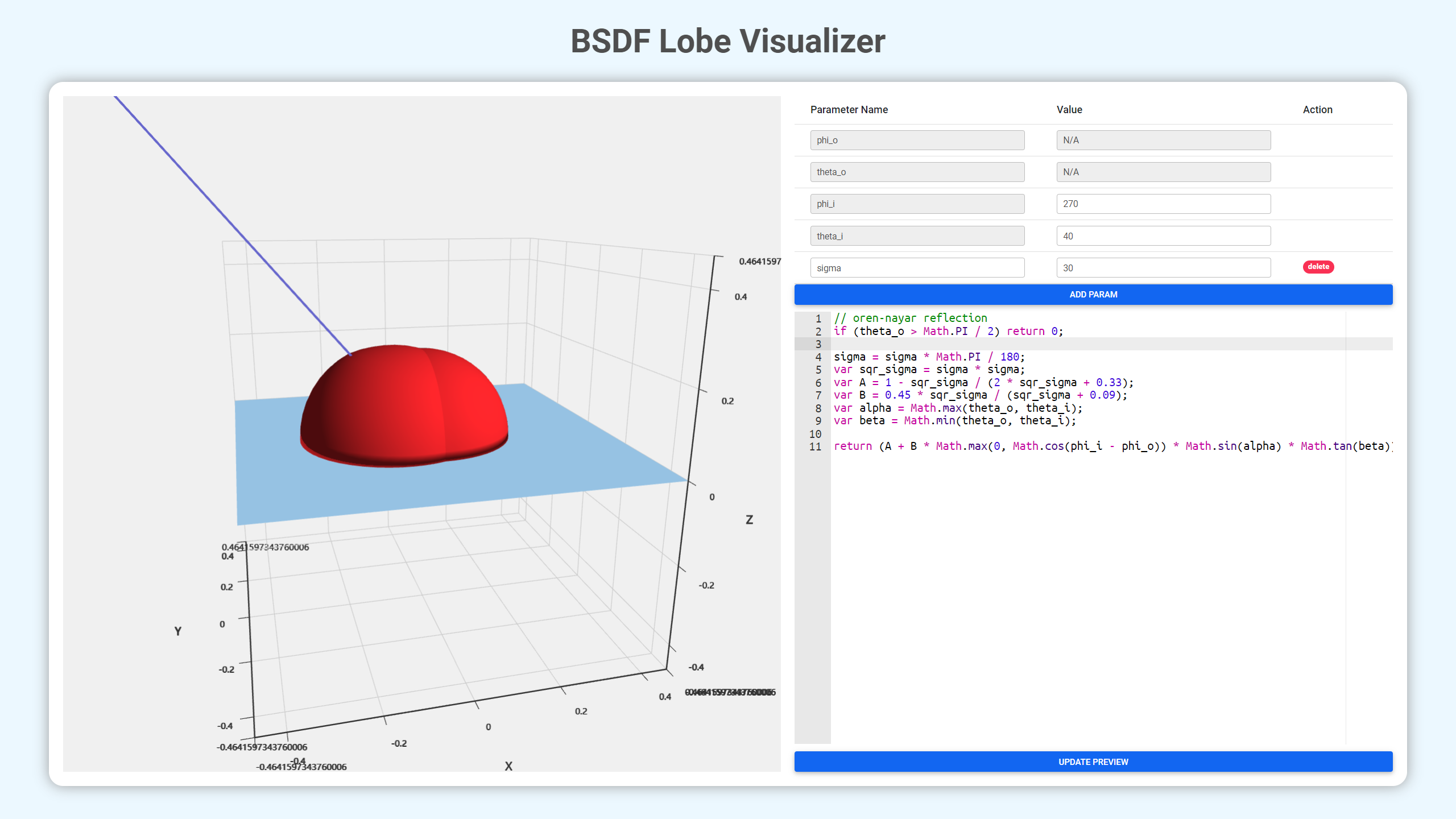# BSDF Lobe 可编程可视化工具 | BSDF Lobe Visualization

\begin{aligned} f_{r, s}\left(\theta_{i}, \varphi_{i}, \theta_{r}, \varphi_{r}, \lambda\right)=&\frac{132 F\left(\theta_{i}, \lambda\right)}{\pi \cos \theta_{i}} \cdot \exp \left[-\left(4 \pi \frac{\sigma}{\lambda} \cos \theta_{i}\right)^{2}\right]+ \\ &\frac{F\left(\theta_{i}, \lambda\right)}{\cos \theta_{i} \cos \theta_{r}} \pi^{3}\left(\frac{a}{\lambda}\right)^{2}\left(\frac{\sigma}{\lambda}\right)^{2}\left(\cos \theta_{i}+\cos \theta_{r}\right)^{4}\cdot \\ & \exp \left\{-\left(\frac{\pi a}{\lambda}\right)^{2}\left[\sin ^{2} \theta_{i}+\sin ^{2} \theta_{r}+2 \sin \theta_{i} \sin \theta_{r} \cos \left(\varphi_{i}-\varphi_{r}\right)\right]\right\} \end{aligned}## 技术路线

（这点屁技术真的有人看得上吗）

• Vue：web 框架
• Material Design Bootstrap：直接嫖的样式
• Ace：代码高亮
• ECharts / ECharts GL：无脑的可视化工具

## 说是 TODO 实际上做不做完全随缘的列表

• [ ] 增加内置的 utils 函数
• [ ] 增加一点 samples
• [ ] 改进 tooltip 的显示内容